# 货币对形态测试: 实际应用和真实交易视角。 第四部分

3 九月 2018, 08:34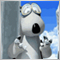0
2 838

### 概述

• 当货币对篮子的统合指标图型与其中之一货币对自身的移动平均线交叉时，交易者会在蜡烛收盘时收到一个进场信号。 有两个这样的信号 — 移动平均线下 穿(卖出) 和上穿 (买入)。

### 研究形态

 我们正在寻找的 "良好" 形态 (信号) 无形态, "坏" 信号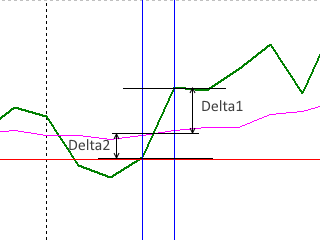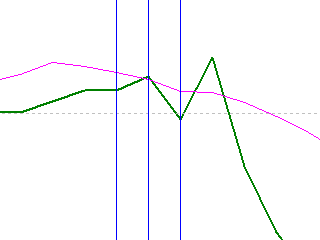### 研究工具

```//+------------------------------------------------------------------+
//|                                  版权所有 2017, MetaQuotes 软件公司 |
//|                                             https://www.mql5.com |
//+------------------------------------------------------------------+
#property copyright "版权所有 2017, MetaQuotes 软件公司"
#property version   "1.00"

//+------------------------------------------------------------------+
//|                                                                  |
//+------------------------------------------------------------------+
enum BSTATE
{
BCLOSE = 0,
BSELL  = 2
};
//+------------------------------------------------------------------+
//| 智能系统初始化函数                                                  |
//+------------------------------------------------------------------+
input int wpr = 20;
input int ma  = 10;
input double lt=0.01;

int h;
ulong  Ticket;

double m,ml;
double w,wl;

BSTATE g_state;

double g_dMinSize = 2.0;
double g_dMaxSize = 50.0;
//+------------------------------------------------------------------+
//|                                                                  |
//+------------------------------------------------------------------+
int OnInit()
{
h=iCustom(NULL,0,"testWPR&MA3",wpr,ma);
if(h==INVALID_HANDLE)
{
Print("Error while creating testWPR&MA3");
return (INIT_FAILED);
}

g_state=BCLOSE;
EventSetTimer(1);
//---
return(INIT_SUCCEEDED);
}
//+------------------------------------------------------------------+
//| 智能系统逆初函数                                                    |
//+------------------------------------------------------------------+
void OnDeinit(const int reason)
{
IndicatorRelease(h);
EventKillTimer();

}
//+------------------------------------------------------------------+
//| 智能系统逐笔报价函数                                                 |
//+------------------------------------------------------------------+
void OnTick()
{

}
//+------------------------------------------------------------------+
//| 定时器函数                                                         |
//+------------------------------------------------------------------+
void OnTimer()
{
if(IsNewCandle())
{
wl=w;
CopyBuffer(h,0,1,1,w);
ml=m;
CopyBuffer(h,1,1,1,m);
double d1 = MathAbs(w - m);
double d2 = MathAbs(ml - wl);
if(w>m && wl<ml)
{
if(g_state!=BCLOSE) CloseAllPos();
if(d1 >= g_dMinSize && d2 >= g_dMinSize &&
d1 <= g_dMaxSize && d2 <= g_dMaxSize)
{
}
}
if(w<m && wl>ml)
{
if(g_state!=BCLOSE) CloseAllPos();
if(d1 >= g_dMinSize && d2 >= g_dMinSize &&
d1 <= g_dMaxSize && d2 <= g_dMaxSize)
{
EnterSell(lt);
g_state=BSELL;
}
}
}
}
//+------------------------------------------------------------------+

void CloseAllPos()
{

g_state=BCLOSE;

}
//+------------------------------------------------------------------+
//|                                                                  |
//+------------------------------------------------------------------+
{

}
//+------------------------------------------------------------------+
//|                                                                  |
//+------------------------------------------------------------------+
void EnterSell(double lot)
{

g_state=BSELL;
}
//+------------------------------------------------------------------+
//|                                                                  |
//+------------------------------------------------------------------+
bool IsNewCandle()
{
static int candle=-1;
int t1=0;
switch(_Period)
{
case PERIOD_H1:  t1 = Hour();   break;
case PERIOD_H4:  t1 = Hour4();  break;
case PERIOD_D1:  t1 = Day();    break;
}

if(t1!=candle) {candle=t1; return(true);}
return (false);
}

int Hour4(){return((int)Hour()/4);}

int Day()
{
MqlDateTime tm;
TimeCurrent(tm);
return(tm.day);
}

int Hour()
{
MqlDateTime tm;
TimeCurrent(tm);
return(tm.hour);
}

```

```//+------------------------------------------------------------------+
//|                                                      testWPR.mq5 |
//|                                               MetaQuotes 软件公司 |
//|                                               http://fxstill.com |
//+------------------------------------------------------------------+
#property copyright "版权所有 2016, MetaQuotes 软件公司"
#property version   "1.00"
#property indicator_separate_window
#property indicator_minimum -100
#property indicator_maximum 100

#property indicator_buffers 2
#property indicator_plots   2

input int WPR       = 20; // WPR 周期
input int maperiod  = 10; // MA 周期
input color   clr   = clrGreen;
input color   clrMA = clrMagenta;

int h,h1;
double ind[],ma[];

//+------------------------------------------------------------------+
//| 自定义指标初始化函数                                                 |
//+------------------------------------------------------------------+
int OnInit()
{
//--- 指标缓存区映射
h = iCustom(NULL,0,"testWPReur",WPR);
if (h == INVALID_HANDLE) {
Print("Error while creating testWPR");
return (INIT_FAILED);
}
h1 = iCustom(NULL,0,"testWPRusd",WPR);
if (h1 == INVALID_HANDLE) {
Print("Error while creating testWPR");
return (INIT_FAILED);
}

IndicatorSetString(INDICATOR_SHORTNAME,"testWPRusd");
IndicatorSetInteger(INDICATOR_DIGITS,2);
IndicatorSetInteger(INDICATOR_LEVELS,2);
IndicatorSetInteger(INDICATOR_LEVELSTYLE,0,STYLE_SOLID);
IndicatorSetInteger(INDICATOR_LEVELSTYLE,1,STYLE_SOLID);
IndicatorSetInteger(INDICATOR_LEVELCOLOR,0,clrRed);
IndicatorSetInteger(INDICATOR_LEVELCOLOR,1,clrRed);
IndicatorSetInteger(INDICATOR_LEVELWIDTH,0,1);
IndicatorSetInteger(INDICATOR_LEVELWIDTH,1,1);
IndicatorSetDouble(INDICATOR_LEVELVALUE,0,-60);
IndicatorSetDouble(INDICATOR_LEVELVALUE,1,60);

ArraySetAsSeries(ind,true);
SetIndexBuffer(0,ind);
PlotIndexSetInteger(0,PLOT_DRAW_TYPE,DRAW_LINE);
PlotIndexSetInteger(0,PLOT_LINE_STYLE,STYLE_SOLID);
PlotIndexSetInteger(0,PLOT_LINE_WIDTH,2);
PlotIndexSetInteger(0,PLOT_LINE_COLOR,clr);
PlotIndexSetString(0,PLOT_LABEL,"_tstWPRusd_");

ArraySetAsSeries(ma,true);
SetIndexBuffer(1,ma);
PlotIndexSetInteger(1,PLOT_DRAW_TYPE,DRAW_LINE);
PlotIndexSetInteger(1,PLOT_LINE_STYLE,STYLE_SOLID);
PlotIndexSetInteger(1,PLOT_LINE_WIDTH,1);
PlotIndexSetInteger(1,PLOT_LINE_COLOR,clrMA);

//---
return(INIT_SUCCEEDED);
}
//+------------------------------------------------------------------+
//|                                                                  |
//+------------------------------------------------------------------+
double GetValue(int shift)
{
double dBuf, dBuf1;
double res=0.0;
CopyBuffer(h,0,shift,1,dBuf);
CopyBuffer(h1,0,shift,1,dBuf1);
return (NormalizeDouble((dBuf - dBuf1)/2, _Digits) );
}
//+------------------------------------------------------------------+
//| 自定义指标迭代函数                                                  |
//+------------------------------------------------------------------+
int OnCalculate(const int rates_total,
const int prev_calculated,
const datetime &time[],
const double &open[],
const double &high[],
const double &low[],
const double &close[],
const long &tick_volume[],
const long &volume[],
{
//---
if(prev_calculated==0 || rates_total>prev_calculated+1)
{
int rt=rates_total-WPR;
for(int i=1; i<rt; i++)
{
ind[i]=GetValue(i);
}
rt-=maperiod;
for(int i=1; i<rt; i++)
{
ma[i]=GetMA(ind,i,maperiod,_Digits);
}
}
else
{
ind = GetValue(0);
ma  = GetMA(ind, 0, maperiod, _Digits);
}
//--- 返回 prev_calculated 的值用于下次调用
return(rates_total);
}
//+------------------------------------------------------------------+

void OnDeinit(const int reason)
{
if(h!=INVALID_HANDLE) IndicatorRelease(h);
if(h1!=INVALID_HANDLE) IndicatorRelease(h1);
}
//+------------------------------------------------------------------+

double GetMA(const double &arr[],int index,int period,int digit)
{
double m=0;
for(int j=0; j<period; j++) m+=arr[index+j];
m/=period;
return (NormalizeDouble(m,digit));
}
//+------------------------------------------------------------------+

```

• 在市场持续存在。 交易者依据信号入场，并等待反方向的进场信号。 然后他们将开单平仓，并在相反方向入场，等等。 我们不会坚守这个策略，因为它 表现得 效率低下。
• 离场而不开新单。 交易者依据信号入场，并等待 "坏" 形态的离场信号。 它不提供入场信号，但它能在趋势放缓或逆转时发出警告。 我们将使用此策略进行测试。

### 测试形态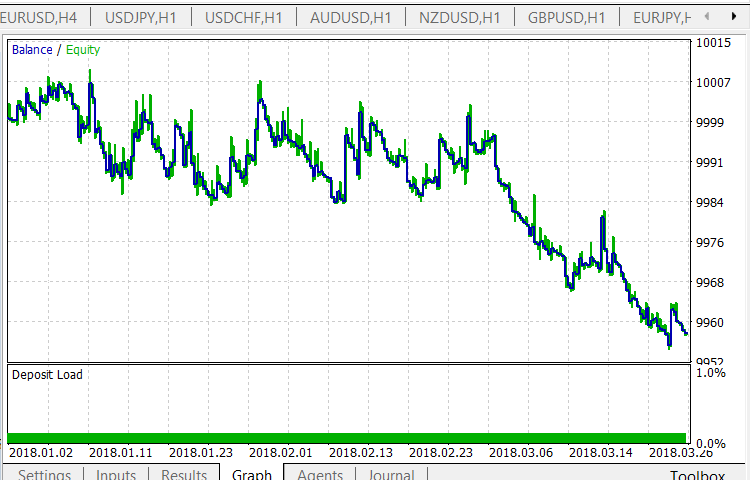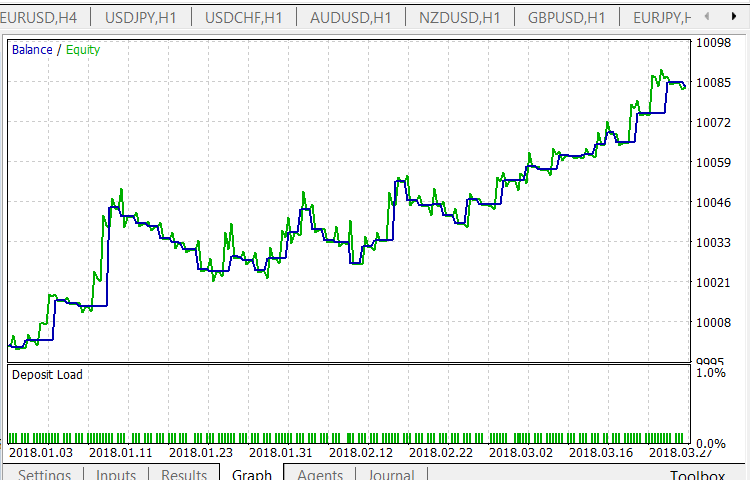• 如果合并的 WPR 图型触及或突破超买边界向上，交易者不要做多。
• 如果合并的 WPR 图型触及或突破超卖边界向下，交易者不要做空。

```//+------------------------------------------------------------------+
//| 定时器函数                                                         |
//+------------------------------------------------------------------+
void OnTimer()
{
if(IsNewCandle())
{
wl=w;
CopyBuffer(h,0,1,1,w);
ml=m;
CopyBuffer(h,1,1,1,m);
double d1 = MathAbs(w - m);
double d2 = MathAbs(ml - wl);
if(w>m && wl<ml)
{
if(g_state!=BCLOSE) CloseAllPos();
if(d1 >= g_dMinSize && d2 >= g_dMinSize &&
d1 <= g_dMaxSize && d2 <= g_dMaxSize && w < 60)
{
}
}
if(w<m && wl>ml)
{
if(g_state!=BCLOSE) CloseAllPos();
if(d1 >= g_dMinSize && d2 >= g_dMinSize &&
d1 <= g_dMaxSize && d2 <= g_dMaxSize && w > -60)
{
EnterSell(lt);
g_state=BSELL;
}
}
}
}
//+------------------------------------------------------------------+
```

• 组合的 WPR 图型突破了超卖级别向上。 在蜡烛收盘时突破仍然存在。 只有当移动平均线 "低于" 组合指标图型时，交易者才会做多。
• 对于做空，情况应该相反。
• 当组合 WPR 图型达到 0％ 时，交易者应离场。

```//+------------------------------------------------------------------+
//|                                  版权所有 2017, MetaQuotes 软件公司 |
//|                                             https://www.mql5.com |
//+------------------------------------------------------------------+
#property copyright "版权所有 2017, MetaQuotes 软件公司"
#property version   "1.00"

enum BSTATE
{
BCLOSE = 0,
BSELL  = 2
};
//+------------------------------------------------------------------+
//| 智能系统初始化函数                                                  |
//+------------------------------------------------------------------+
input int wpr = 20;
input int ma  = 10;
input double lt=0.01; //手数

int SELLPROFIT =   0;
int SELL1LIMIT =  50;
int SELL2FROM  =  40;
int SELL2TO    =  20;

int h;
ulong  Ticket;

double m,ml;
double w,wl;

BSTATE g_state;

double g_dMinSize = 2.0;
double g_dMaxSize = 50.0;
//+------------------------------------------------------------------+
//|                                                                  |
//+------------------------------------------------------------------+
int OnInit()
{
h=iCustom(NULL,0,"testWPR&MA3",wpr,ma);
if(h==INVALID_HANDLE)
{
Print("Error while creating testWPR&MA3");
return (INIT_FAILED);
}

g_state=BCLOSE;
EventSetTimer(1);
//---
return(INIT_SUCCEEDED);
}
//+------------------------------------------------------------------+
//| 智能系统逆初函数                                                    |
//+------------------------------------------------------------------+
void OnDeinit(const int reason)
{
IndicatorRelease(h);
EventKillTimer();

}
//+------------------------------------------------------------------+
//| 智能系统逐笔报价函数                                                 |
//+------------------------------------------------------------------+
void OnTick()
{

}
//+------------------------------------------------------------------+
//| 定时器函数                                                         |
//+------------------------------------------------------------------+
void OnTimer()
{
if(IsNewCandle())
{
wl=w;
CopyBuffer(h,0,1,1,w);
ml=m;
CopyBuffer(h,1,1,1,m);

{
CloseAllPos();
}
if(g_state==BSELL && (w<=SELLPROFIT))
{
CloseAllPos();
}
{
return;
}

if(g_state==BCLOSE && w<=SELL2FROM && w>=SELL2TO && wl>=SELL1LIMIT && w < m)
{
EnterSell(lt);
return;
}
}
}
//+------------------------------------------------------------------+

void CloseAllPos()
{

g_state=BCLOSE;

}
//+------------------------------------------------------------------+
//|                                                                  |
//+------------------------------------------------------------------+
{

}
//+------------------------------------------------------------------+
//|                                                                  |
//+------------------------------------------------------------------+
void EnterSell(double lot)
{

g_state=BSELL;
}
//+------------------------------------------------------------------+
//|                                                                  |
//+------------------------------------------------------------------+
bool IsNewCandle()
{
static int candle=-1;
int t1=0;
switch(_Period)
{
case PERIOD_H1:  t1 = Hour();   break;
case PERIOD_H4:  t1 = Hour4();  break;
case PERIOD_D1:  t1 = Day();    break;
}

if(t1!=candle) {candle=t1; return(true);}
return (false);
}

int Hour4(){return((int)Hour()/4);}

int Day()
{
MqlDateTime tm;
TimeCurrent(tm);
return(tm.day);
}

int Hour()
{
MqlDateTime tm;
TimeCurrent(tm);
return(tm.hour);
}

```

H1 时间帧的结果: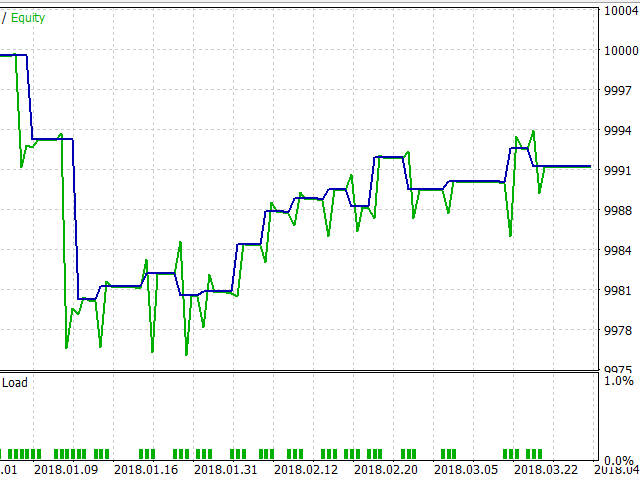H4 时间帧的结果: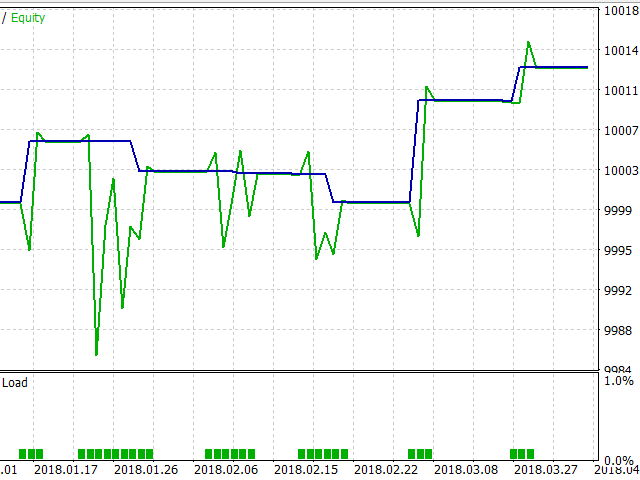### 结果

1. 我们已研究过在交易中使用货币对篮子的方法。 已提供了基本原理和概念，以及基本计算类型。
2. 在交易货币对篮子时会出现的若干种简单形态也已描述过了。
3. 使用篮子时出现的更复杂结构已也简要研究 — 同时使用标准技术指标和专门为货币对设计的指标; 同时使用振荡器和趋势跟踪指标。
4. 查验已检测到的形态效率。

### 实际交易中的前景与应用

#### 入场/离场详情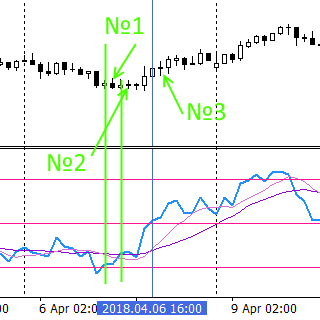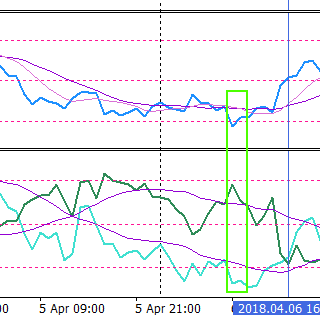Indicator (NZDUSD) = Indicator (NZD) — Indicator (USD)

1. 较低的红色水平线是超卖等级。 我们可以看到，指标图型的形态首先形成了突破这一等级。 这个位置用垂直绿线标记，而形态本身在蜡烛 1 开盘时被识别。 此刻，您可开多头单: 下跌趋势已经耗尽其能量，并开始逆转。 交易者捕捉到这一走势的开始，并能捕捉到整个上升趋势。 我们已测试了这种形态。 这是相当危险的 (因为下跌趋势可能在调整后继续)，且很少出现。 在这种情况下，交易者的潜在亏损并不太多。 这里可以使用较短的止损。
2. 然而，在屏幕截图中，我们看到新的上升趋势开始获得动力。 新形态正在形成 — 指标图型突破了移动平均线。 超卖等级被突破之后，这是一个良好的信号滤波器。 它降低了下跌趋势持续的风险。 该形态还标有绿色垂直线，并可以在蜡烛 2 的开盘处识别。 您也可以在此刻入场。 通常，止损应该更大 (由移动平均线参数定义)。
3. 在上升趋势增强后，出现一个新形态 — 快速均线突破慢速均线。 这种形态在蜡烛 3 的开盘处被识别。 与前两种情况相比，风险很小。 但是，盈利能力较低，而止损较大。 注意在检测到形态后移动平均线之间的距离如何增加。 这示意趋势变得更强 — 这对交易者来说是一个有利的信号。 距离的减小表示减速和减弱 (参见左侧屏幕截图)。

• 在右侧屏幕截图中，我们可以看到指标图型如何进入超卖 (或超买) 区域。 必要的地方用绿色矩形标记。 在指标窗口中，相同的指标以另一种方式显示 — 分别用于 NZD (浅蓝色线) 和 USD (深绿色线) 篮子。 我们可以看到 USD 篮子在某些时候变得超买，而 NZD 则在很长一段时间内超卖。 篮子的这些状态组合的的同时构成了一幅图型，如左侧屏幕截图所示，NZDUSD 的组合指标图型进入超买区域，随后突破超买等级并趋势反转。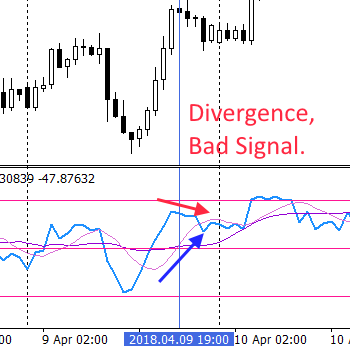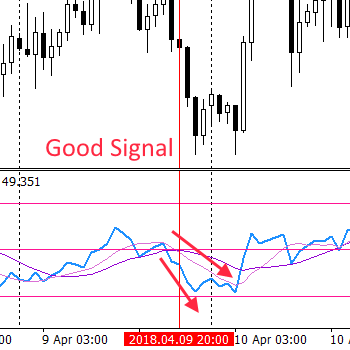1. 例如，指标进入超卖区域 — 这是多头持仓平仓或将其移至盈亏平衡的显要原因 (空头持仓相反)。

2. 交易者可检测与手中持仓方向相反的均线突破指标图型的信号。

3. 交易者可通过快速和慢速均线间的距离减小来检测趋势正在减缓。

4. 最后，依照指标触及非超买/超卖等级的数值，作为平仓或/移动到盈亏平衡的信号。 例如, 这也许是 等级 0%。 这一级别意味着两种货币篮子的 "相等"。 指标往往在这样的等级上 "徘徊"。 因此，这个等级是一个阻力: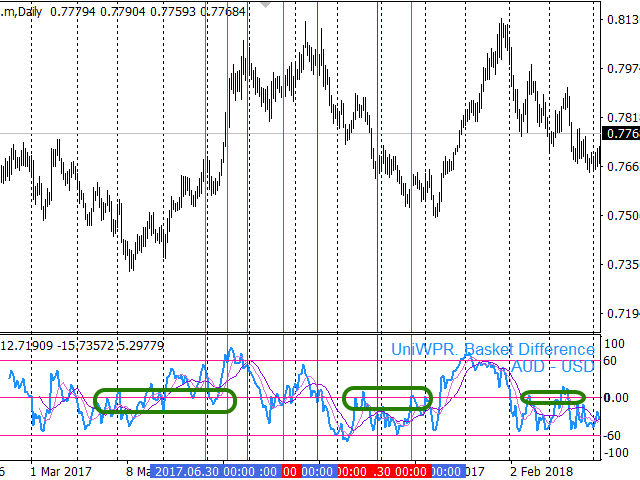5. 最后一件事: 交易者可以根据自己的考量或标准技术分析来分配止损和止盈。

#### 使用统合指标操作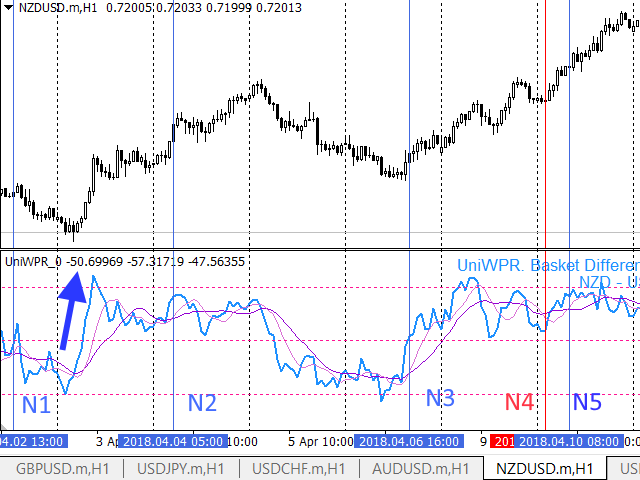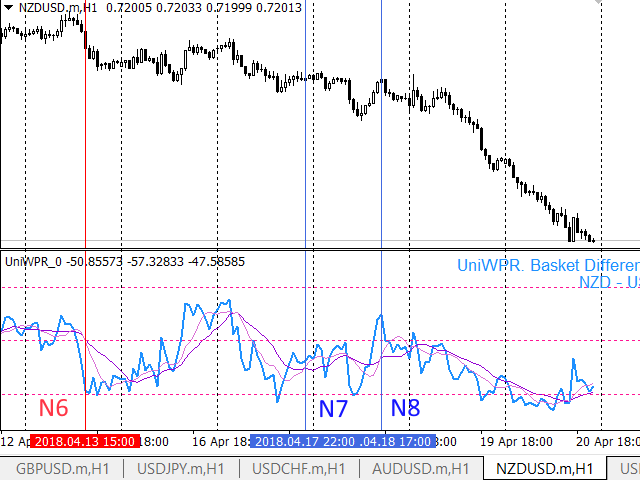#### 组合指标的高级操作

• 该图表包含两个具有相似设置，但显示方法不同的组合指标的实例:
1. 默认为 V1 窗口。
2. 基准和报价货币篮子的两个单独图表 (V2 窗口)。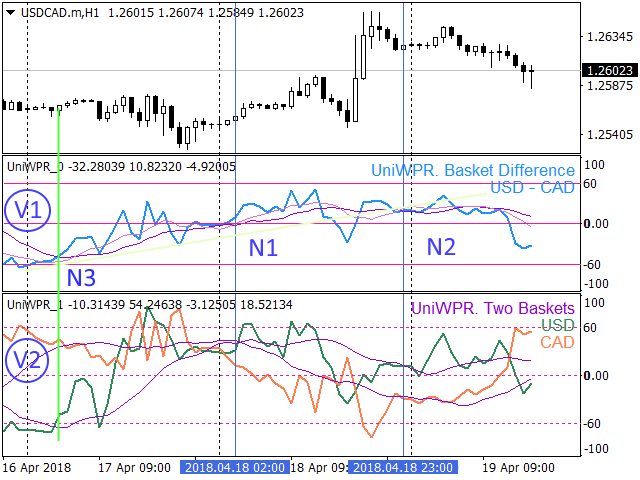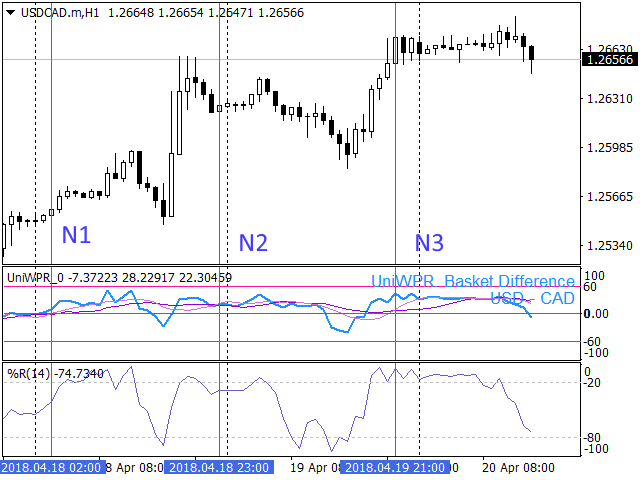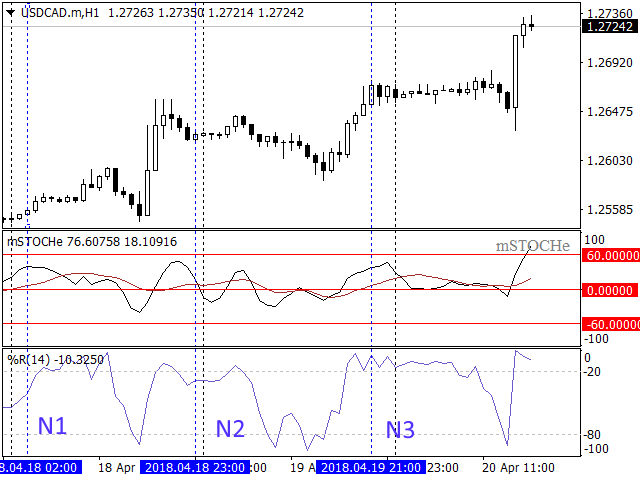### 本文中使用的程序

# 名称

描述
1 test.zip 存档

2
testhtml.zip 存档 来自 test.zip 存档的使用工具进行测试的结果
3 test1.zip 存档 含过滤器的测试 EA 代码
4 testhtml50.zip 存档 来自 test1.zip 存档的使用工具进行测试的结果
5
testhtml60.zip 存档

test.zip (15.54 KB)
testhtml.zip (1197.56 KB)
testhtml50.zip (226.91 KB)
testhtml60.zip (200.84 KB)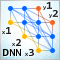深度神经网络 (第七部分)。 神经网络的融合: 堆叠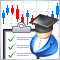如何创建订购交易机器人的需求规范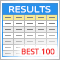可视化使用选定标准优化的结果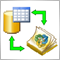整合基于MQL的EA交易和数据库 (SQL Server, .NET 和 C#)